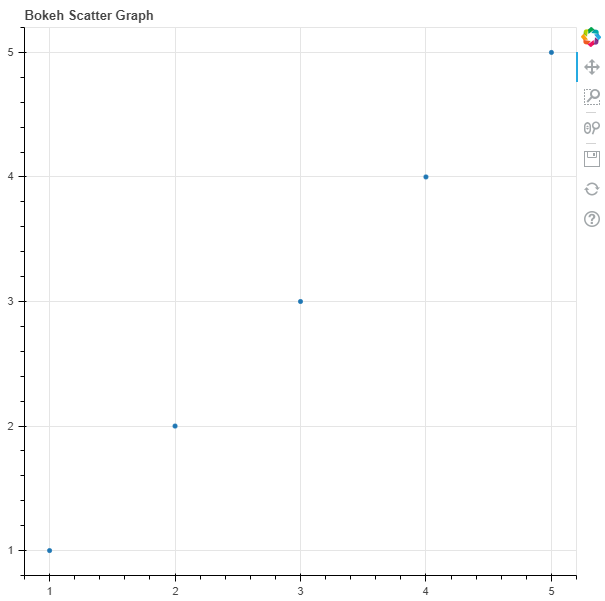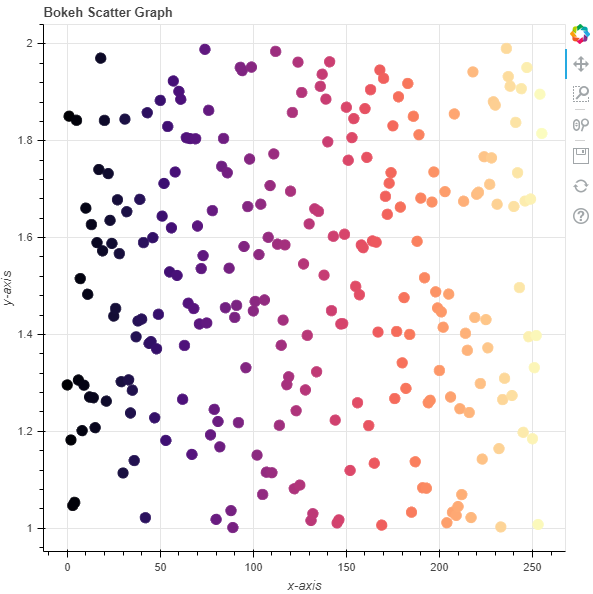# Python Bokeh – Plotting a Scatter Plot on a Graph

• Last Updated : 10 Jul, 2020

Bokeh is a Python interactive data visualization. It renders its plots using HTML and JavaScript. It targets modern web browsers for presentation providing elegant, concise construction of novel graphics with high-performance interactivity.

Bokeh can be used to plot a scatter plot on a graph. Plotting squares on a graph can be done using the `scatter()` method of the `plotting` module.

## plotting.figure.scatter()

Syntax : scatter(parameters)

Parameters :

• x : x-coordinates of the center of the glyphs
• y : y-coordinates of the center of the glyphs
• marker : signifies the type of the glyph

Returns : an object of class `GlyphRenderer`

Example 1 : In this example we will be using the default values for plotting the graph.

 `# importing the modules``from` `bokeh.plotting ``import` `figure, output_file, show``       ` `# file to save the model``output_file(``"gfg.html"``)``       ` `# instantiating the figure object``graph ``=` `figure(title ``=` `"Bokeh Scatter Graph"``)``     ` `# the points to be plotted``x ``=` `[``1``, ``2``, ``3``, ``4``, ``5``]``y ``=` `[``1``, ``2``, ``3``, ``4``, ``5``]``    ` `# plotting the graph``graph.scatter(x, y)``     ` `# displaying the model``show(graph)`

Output :Example 2 : In this example we will be plotting multiple scatter points with various other parameters

 `# importing the modules ``from` `bokeh.plotting ``import` `figure, output_file, show ``from` `bokeh.palettes ``import` `magma``import` `random`` ` `# file to save the model ``output_file(``"gfg.html"``) ``      ` `# instantiating the figure object ``graph ``=` `figure(title ``=` `"Bokeh Scatter Graph"``) `` ` `# name of the x-axis ``graph.xaxis.axis_label ``=` `"x-axis"``      ` `# name of the y-axis ``graph.yaxis.axis_label ``=` `"y-axis"`` ` `# points to be plotted``x ``=` `[n ``for` `n ``in` `range``(``256``)]``y ``=` `[random.random() ``+` `1` `for` `n ``in` `range``(``256``)]``size ``=` `10`` ` `# color value of the scatter points``color ``=` `magma(``256``)`` ` `# plotting the graph ``graph.scatter(x, y,``              ``size ``=` `size,``              ``color ``=` `color) ``      ` `# displaying the model ``show(graph)`

Output :My Personal Notes arrow_drop_up